•5星
1.32MB weixin_44573410 2021-05-26 22:17:12
•5星
2KB wenyusuran 2021-02-14 21:47:21
•5星
2.28MB qq_40957277 2021-06-22 18:53:14
• 前向传播 深度学习 机器学习 神经网络

好，下面开始看看啥是前向传播 神经网络实际上就是一个输入为x,输出为y的映射函数 前向传播就是给网络输入一个样本向量，该样本向量的各元素，经过各隐藏层的逐级加权求和+非线性激活，最终由输出层输出一个预测...

我希望自己也是一颗星星。如果我会发光，就不必害怕黑暗。
如果我自己是那么美好，那么一切恐惧就可以烟消云散。
于是我开始存下了一点希望。——王小波

下面所有内容均来自网络和自己的一点点理解。（侵删）

额，它好像是属于神经网络的知识，so我先查了一下啥是神经网络

神经网络可以指向两种，一是生物神经网络，一是人工神经网络
生物神经网络：一般指生物的大脑神经元，细胞，触点等组成的网络，用于产生生物的意识，帮助生物进行思考和行动。
人工神经网络（Artificial Neural Networks，简写为ANNs）也简称为神经网络（NNs）或称作连接模型（Connection Model），它是一种模仿动物神经网络行为特征，进行分布式并行信息处理的算法数学模型。这种网络依靠系统的复杂程度，通过调整内部大量节点之间相互连接的关系，从而达到处理信息的目的。

大概有一点明白了，就是一种算法模型对吧，关键词抓取：分布式、内部大量结点之间相互连接

在机器学习和相关领域，人工神经网络（人工神经网络）的计算模型灵感来自动物的中枢神经系统（尤其是脑），并且被用于估计或可以依赖于大量的输入和一般的未知近似函数。人工神经网络通常呈现为相互连接的“神经元”，它可以从输入的计算值，并且能够机器学习以及模式识别由于它们的自适应性质的系统。
例如，用于手写体识别的神经网络是由一组可能被输入图像的像素激活的输入神经元来限定。后进过加权，并通过一个函数（由网络的设计者确定的）转化，这些神经元的致动被上到其他神经元然后被传递。重复此过程，直到最后，一输出神经元被激活。这决定了哪些字符被读取。
像其他的从数据-神经网络认识到的机器学习系统方法已被用来解决各种各样的很难用普通的以规则为基础的编程解决的任务，包括计算机视觉和语音识别。
也许，人工神经网络的最大优势是他们能够被用作一个任意函数逼近的机制，那是从观测到的数据“学习”。然而，使用起来也不是那么简单的，一个比较好理解的基本理论是必不可少的。

好，下面开始看看啥是前向传播

神经网络实际上就是一个输入为x,输出为y的映射函数
前向传播就是给网络输入一个样本向量，该样本向量的各元素，经过各隐藏层的逐级加权求和+非线性激活，最终由输出层输出一个预测向量的过程

然后我又去查了啥是加权求和：

就是根据权重比求和（就是比如我们学校高数的最终分数是根据平时作业，月考，期中考，期末考四项决定的，而这四项对最终成绩影响不同，期末成绩占70%）

啥又是非线性激活？

这就要先知道啥是线性激活函数了。。。

a=z 叫做线性激活函数 或 恒等激活函数
无论你有多少个隐含层，只要是使用线性激活函数，一直做的就只是计算线性激活函数（这就是最原始的感知机）
只有一个地方可以使用线性激活函数，那就是回归问题。或者在输出层用也是可以的。

非线性函数就是:（我在网上搜了好久啥是非线性激活函数，怎么都查不到。。。没想要拆开来查就能查到了）

图像不是一条直线的函数

激活函数：

它们将非线性特性引入到我们的网络中。比如：在神经元中，输入的 inputs 通过加权，求和后，还被作用了一个函数，这个函数就是激活函数。
引入激活函数是为了增加神经网络模型的非线性。没有激活函数的每层都相当于矩阵相乘。就算你叠加了若干层之后，无非还是个矩阵相乘罢了。

前向传播和反向传播模拟的是人脑中神经元的正向传导和反向反馈信号回路
前向传播输入信号直至输出产生误差，反向传播误差更新权重矩阵。

还有好多常用的激活函数（全来自百度哈）
Sigmoid函数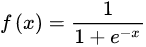图像：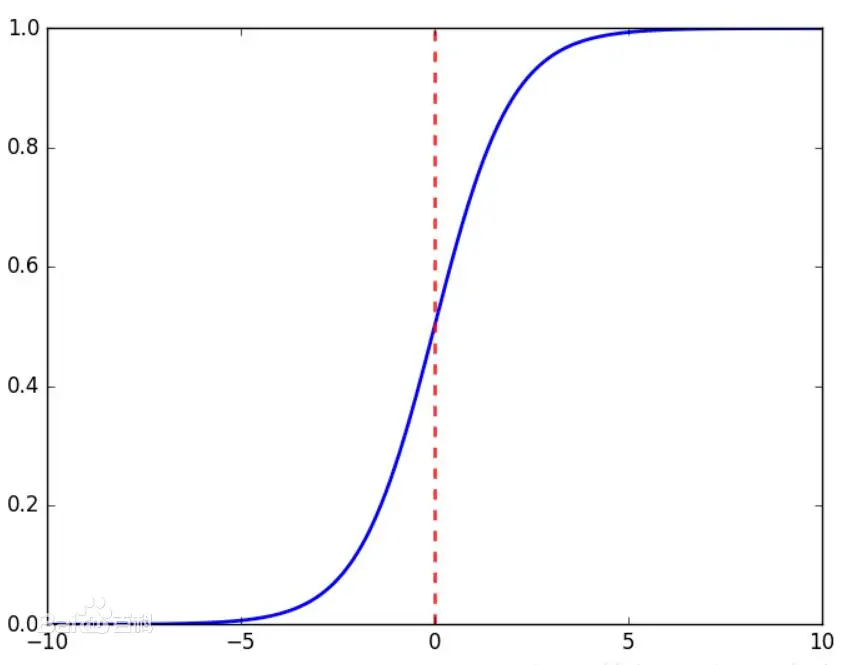Tanh函数
Tanh是双曲函数中的一个，Tanh()为双曲正切。（在数学中，双曲正切“Tanh”是由基本双曲函数双曲正弦和双曲余弦推导而来。）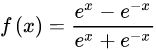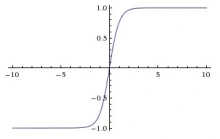ReLU函数
Relu激活函数（The Rectified Linear Unit），用于隐层神经元输出。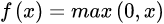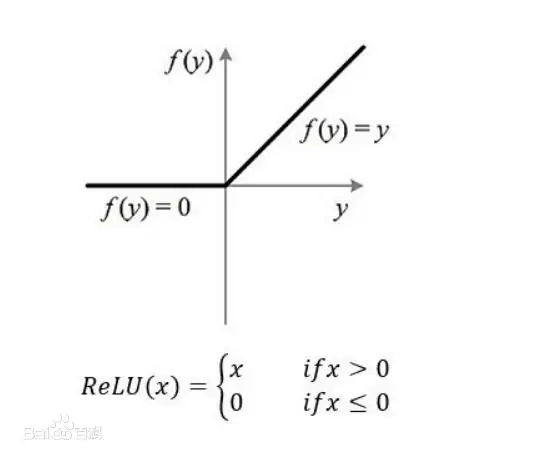啥是隐层神经元啊？（来自csdn的n篇文章）

要说明隐藏层的意义，需要从两个方面理解，一个是单个隐藏层的意义，一个是多层隐藏层的意义。

单个隐藏层的意义就是把输入数据的特征，抽象到另一个维度空间，来展现其更抽象化的特征，这些特征能更好的进行线性划分。

多个隐藏层其实是对输入特征多层次的抽象，最终的目的就是为了更好的线性划分不同类型的数据（不是层数越多越好的）

层数越多参数会爆炸式增多
到了一定层数，再往深了加隐藏层，分类效果的增强会越来越不明显。

展开全文misakisaigao 2021-04-14 19:13:51
• TensorFlow2 手把手教你实现前向传播.

概述

前向传播 (Forward propagation) 是将上一层输出作为下一层的输入, 并计算下一层的输出, 一直到运算到输出层为止.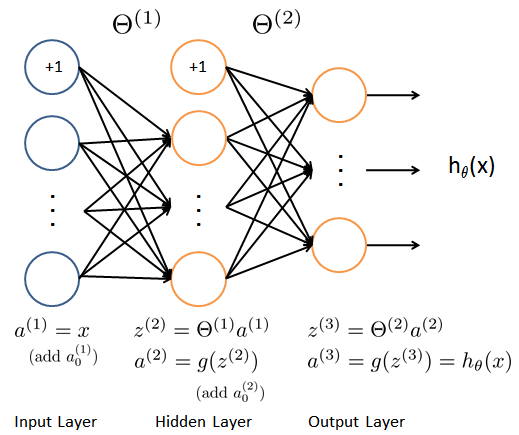会用到的函数张量最小值

reduce_min函数可以帮助我们计算一个张量各个维度上元素的最小值.

格式:

tf.math.reduce_min(
input_tensor, axis=None, keepdims=False, name=None
)

参数:

• input_tensor: 传入的张量
• axis: 维度, 默认计算所有维度
• keepdims: 如果为真保留维度, 默认为 False
• name: 数据名称

张量最大值

reduce_max函数可以帮助我们计算一个张量各个维度上元素的最大值.

格式:

tf.math.reduce_max(
input_tensor, axis=None, keepdims=False, name=None
)

参数:

• input_tensor: 传入的张量
• axis: 维度, 默认计算所有维度
• keepdims: 如果为真保留维度, 默认为 False
• name: 数据名称

数据集分批

from_tensor_slices可以帮助我们切分传入 Tensor 的第一个维度. 得到的每个切片都是一个样本数据.格式:

@staticmethod
from_tensor_slices(
tensors
)

迭代

我们可以调用iter函数来生成迭代器.

格式:

iter(object[, sentinel])

参数:
-object: 支持迭代的集合对象

• sentinel: 如果传递了第二个参数, 则参数 object 必须是一个可调用的对象 (如, 函数). 此时, iter 创建了一个迭代器对象, 每次调用这个迭代器对象的__next__()方法时, 都会调用 object

例子:

list = [1, 2, 3]
i = iter(list)
print(next(i))
print(next(i))
print(next(i))

输出结果:

1
2
3

截断正态分布

truncated_normal可以帮助我们生成一个截断的正态分布. 生成的正态分布值会在两倍的标准差的范围之内.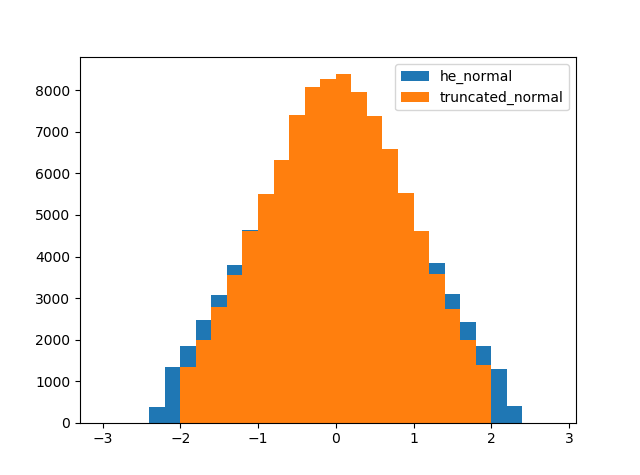格式:

tf.random.truncated_normal(
shape, mean=0.0, stddev=1.0, dtype=tf.dtypes.float32, seed=None, name=None
)

参数:

• shape: 张量的形状
• mean: 正态分布的均值, 默认 0.0
• stddev: 正态分布的标准差, 默认为 1.0
• dtype: 数据类型, 默认为 float32
• seed: 随机数种子
• name: 数据名称

relu 激活函数

激活函数有 sigmoid, maxout, relu 等等函数. 通过激活函数我们可以使得各个层之间达成非线性关系.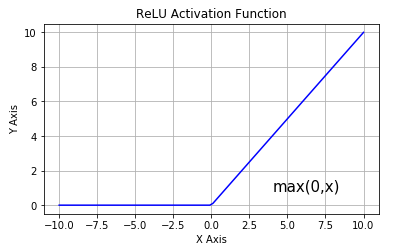激活函数可以帮助我们提高模型健壮性, 提高非线性表达能力, 缓解梯度消失问题.

one_hot

tf.one_hot函数是讲 input 准换为 one_hot 类型数据输出. 相当于将多个数值联合放在一起作为多个相同类型的向量.

格式:

tf.one_hot(
indices, depth, on_value=None, off_value=None, axis=None, dtype=None, name=None
)

参数:

• indices: 索引的张量
• depth: 指定独热编码维度的标量
• on_value: 索引 indices[j] = i 位置处填充的标量，默认为 1
• off_value: 索引 indices[j] != i 所有位置处填充的标量, 默认为 0
• axis: 填充的轴, 默认为 -1 (最里面的新轴)
• dtype: 输出张量的数据格式
• name：数据名称

assign_sub

assign_sub可以帮助我们实现张量自减.

格式:

tf.compat.v1.assign_sub(
ref, value, use_locking=None, name=None
)

参数:

• ref: 多重张量
• value: 张量
• use_locking: 锁
• name: 数据名称

准备工作import tensorflow as tf

# 定义超参数
batch_size = 256  # 一次训练的样本数目
learning_rate = 0.001  # 学习率
iteration_num = 20  # 迭代次数

# 读取mnist数据集
(x, y), _ = tf.keras.datasets.mnist.load_data()  # 读取训练集的特征值和目标值
print(x[:5])  # 调试输出前5个图
print(y[:5])  # 调试输出前5个目标值数字
print(x.shape)  # (60000, 28, 28) 单通道
print(y.shape)  # (60000,)

# 转换成常量tensor
x = tf.convert_to_tensor(x, dtype=tf.float32) / 255  # 转换为0~1的形式
y = tf.convert_to_tensor(y, dtype=tf.int32)  # 转换为整数形式

# 调试输出范围
print(tf.reduce_min(x), tf.reduce_max(x))  # 0~1
print(tf.reduce_min(y), tf.reduce_max(y))  # 0~9

# 分割数据集
train_db = tf.data.Dataset.from_tensor_slices((x, y)).batch(batch_size)  # 256为一个batch
train_iter = iter(train_db)  # 生成迭代对象

# 定义权重和bias [256, 784] => [256, 256] => [256, 128] => [128, 10]
w1 = tf.Variable(tf.random.truncated_normal([784, 256], stddev=0.1))  # 标准差为0.1的截断正态分布
b1 = tf.Variable(tf.zeros())  # 初始化为0

w2 = tf.Variable(tf.random.truncated_normal([256, 128], stddev=0.1))  # 标准差为0.1的截断正态分布
b2 = tf.Variable(tf.zeros())  # 初始化为0

w3 = tf.Variable(tf.random.truncated_normal([128, 10], stddev=0.1))  # 标准差为0.1的截断正态分布
b3 = tf.Variable(tf.zeros())  # 初始化为0

输出结果:

[[[0 0 0 ... 0 0 0]
[0 0 0 ... 0 0 0]
[0 0 0 ... 0 0 0]
...
[0 0 0 ... 0 0 0]
[0 0 0 ... 0 0 0]
[0 0 0 ... 0 0 0]]

[[0 0 0 ... 0 0 0]
[0 0 0 ... 0 0 0]
[0 0 0 ... 0 0 0]
...
[0 0 0 ... 0 0 0]
[0 0 0 ... 0 0 0]
[0 0 0 ... 0 0 0]]

[[0 0 0 ... 0 0 0]
[0 0 0 ... 0 0 0]
[0 0 0 ... 0 0 0]
...
[0 0 0 ... 0 0 0]
[0 0 0 ... 0 0 0]
[0 0 0 ... 0 0 0]]

[[0 0 0 ... 0 0 0]
[0 0 0 ... 0 0 0]
[0 0 0 ... 0 0 0]
...
[0 0 0 ... 0 0 0]
[0 0 0 ... 0 0 0]
[0 0 0 ... 0 0 0]]

[[0 0 0 ... 0 0 0]
[0 0 0 ... 0 0 0]
[0 0 0 ... 0 0 0]
...
[0 0 0 ... 0 0 0]
[0 0 0 ... 0 0 0]
[0 0 0 ... 0 0 0]]]
[5 0 4 1 9]
(60000, 28, 28)
(60000,)
tf.Tensor(0.0, shape=(), dtype=float32) tf.Tensor(1.0, shape=(), dtype=float32)
tf.Tensor(0, shape=(), dtype=int32) tf.Tensor(9, shape=(), dtype=int32)

train 函数

def train(epoch):  # 训练
for step, (x, y) in enumerate(train_db):  # 每一批样本遍历
# 把x平铺 [256, 28, 28] => [256, 784]
x = tf.reshape(x, [-1, 784])

with tf.GradientTape() as tape:  # 自动求解
# 第一个隐层 [256, 784] => [256, 256]
# [256, 784]@[784, 256] +  => [256, 256] +  => [256, 256] + [256, 256] (广播机制)
h1 = x @ w1 + tf.broadcast_to(b1, [x.shape, 256])
h1 = tf.nn.relu(h1)  # relu激活

# 第二个隐层 [256, 256] => [256, 128]
h2 = h1 @ w2 + b2
h2 = tf.nn.relu(h2)  # relu激活

# 输出层 [256, 128] => [128, 10]
out = h2 @ w3 + b3

# 计算损失MSE(Mean Square Error)
y_onehot = tf.one_hot(y, depth=10)  # 转换成one_hot编码
loss = tf.square(y_onehot - out)  # 计算总误差
loss = tf.reduce_mean(loss)  # 计算平均误差MSE

# 计算梯度

# 更新权重

if step % 100 == 0:  # 每运行100个批次, 输出一次
print("epoch:", epoch, "step:", step, "loss:", float(loss))

run 函数

def run():
for i in range(iteration_num):  # 迭代20次
train(i)

完整代码

import tensorflow as tf

# 定义超参数
batch_size = 256  # 一次训练的样本数目
learning_rate = 0.001  # 学习率
iteration_num = 20  # 迭代次数

# 读取mnist数据集
(x, y), _ = tf.keras.datasets.mnist.load_data()  # 读取训练集的特征值和目标值
print(x[:5])  # 调试输出前5个图
print(y[:5])  # 调试输出前5个目标值数字
print(x.shape)  # (60000, 28, 28) 单通道
print(y.shape)  # (60000,)

# 转换成常量tensor
x = tf.convert_to_tensor(x, dtype=tf.float32) / 255  # 转换为0~1的形式
y = tf.convert_to_tensor(y, dtype=tf.int32)  # 转换为整数形式

# 调试输出范围
print(tf.reduce_min(x), tf.reduce_max(x))  # 0~1
print(tf.reduce_min(y), tf.reduce_max(y))  # 0~9

# 分割数据集
train_db = tf.data.Dataset.from_tensor_slices((x, y)).batch(batch_size)  # 256为一个batch
train_iter = iter(train_db)  # 生成迭代对象

# 定义权重和bias [256, 784] => [256, 256] => [256, 128] => [128, 10]
w1 = tf.Variable(tf.random.truncated_normal([784, 256], stddev=0.1))  # 标准差为0.1的截断正态分布
b1 = tf.Variable(tf.zeros())  # 初始化为0

w2 = tf.Variable(tf.random.truncated_normal([256, 128], stddev=0.1))  # 标准差为0.1的截断正态分布
b2 = tf.Variable(tf.zeros())  # 初始化为0

w3 = tf.Variable(tf.random.truncated_normal([128, 10], stddev=0.1))  # 标准差为0.1的截断正态分布
b3 = tf.Variable(tf.zeros())  # 初始化为0

def train(epoch):  # 训练
for step, (x, y) in enumerate(train_db):  # 每一批样本遍历
# 把x平铺 [256, 28, 28] => [256, 784]
x = tf.reshape(x, [-1, 784])

with tf.GradientTape() as tape:  # 自动求解
# 第一个隐层 [256, 784] => [256, 256]
# [256, 784]@[784, 256] +  => [256, 256] +  => [256, 256] + [256, 256] (广播机制)
h1 = x @ w1 + tf.broadcast_to(b1, [x.shape, 256])
h1 = tf.nn.relu(h1)  # relu激活

# 第二个隐层 [256, 256] => [256, 128]
h2 = h1 @ w2 + b2
h2 = tf.nn.relu(h2)  # relu激活

# 输出层 [256, 128] => [128, 10]
out = h2 @ w3 + b3

# 计算损失MSE(Mean Square Error)
y_onehot = tf.one_hot(y, depth=10)  # 转换成one_hot编码
loss = tf.square(y_onehot - out)  # 计算总误差
loss = tf.reduce_mean(loss)  # 计算平均误差MSE

# 计算梯度

# 更新权重

if step % 100 == 0:  # 每运行100个批次, 输出一次
print("epoch:", epoch, "step:", step, "loss:", float(loss))

def run():
for i in range(iteration_num):  # 迭代20次
train(i)

if __name__ == "__main__":
run()

输出结果:

epoch: 0 step: 0 loss: 0.5439826250076294
epoch: 0 step: 100 loss: 0.2263326346874237
epoch: 0 step: 200 loss: 0.19458135962486267
epoch: 1 step: 0 loss: 0.1788959801197052
epoch: 1 step: 100 loss: 0.15782299637794495
epoch: 1 step: 200 loss: 0.1580992043018341
epoch: 2 step: 0 loss: 0.15085121989250183
epoch: 2 step: 100 loss: 0.1432340145111084
epoch: 2 step: 200 loss: 0.14373672008514404
epoch: 3 step: 0 loss: 0.13810500502586365
epoch: 3 step: 100 loss: 0.13337770104408264
epoch: 3 step: 200 loss: 0.1334681361913681
epoch: 4 step: 0 loss: 0.12887853384017944
epoch: 4 step: 100 loss: 0.12551936507225037
epoch: 4 step: 200 loss: 0.125375896692276
epoch: 5 step: 0 loss: 0.12160968780517578
epoch: 5 step: 100 loss: 0.1190723180770874
epoch: 5 step: 200 loss: 0.11880680173635483
epoch: 6 step: 0 loss: 0.11563797295093536
epoch: 6 step: 100 loss: 0.11367204040288925
epoch: 6 step: 200 loss: 0.11331651359796524
epoch: 7 step: 0 loss: 0.11063456535339355
epoch: 7 step: 100 loss: 0.10906648635864258
epoch: 7 step: 200 loss: 0.10866570472717285
epoch: 8 step: 0 loss: 0.10636782646179199
epoch: 8 step: 100 loss: 0.10510052740573883
epoch: 8 step: 200 loss: 0.10468046367168427
epoch: 9 step: 0 loss: 0.10268573462963104
epoch: 9 step: 100 loss: 0.10163718461990356
epoch: 9 step: 200 loss: 0.10121693462133408
epoch: 10 step: 0 loss: 0.09949333965778351
epoch: 10 step: 100 loss: 0.09859145432710648
epoch: 10 step: 200 loss: 0.09819269925355911
epoch: 11 step: 0 loss: 0.0966767817735672
epoch: 11 step: 100 loss: 0.09586615860462189
epoch: 11 step: 200 loss: 0.09550992399454117
epoch: 12 step: 0 loss: 0.09417577087879181
epoch: 12 step: 100 loss: 0.09341947734355927
epoch: 12 step: 200 loss: 0.09310202300548553
epoch: 13 step: 0 loss: 0.09193204343318939
epoch: 13 step: 100 loss: 0.09122277796268463
epoch: 13 step: 200 loss: 0.09092779457569122
epoch: 14 step: 0 loss: 0.0899026170372963
epoch: 14 step: 100 loss: 0.08923697471618652
epoch: 14 step: 200 loss: 0.08895798027515411
epoch: 15 step: 0 loss: 0.08804921805858612
epoch: 15 step: 100 loss: 0.08742769062519073
epoch: 15 step: 200 loss: 0.0871589332818985
epoch: 16 step: 0 loss: 0.08635203540325165
epoch: 16 step: 100 loss: 0.0857706069946289
epoch: 16 step: 200 loss: 0.0855005756020546
epoch: 17 step: 0 loss: 0.08479145169258118
epoch: 17 step: 100 loss: 0.08423925191164017
epoch: 17 step: 200 loss: 0.08396687358617783
epoch: 18 step: 0 loss: 0.08334997296333313
epoch: 18 step: 100 loss: 0.08281457424163818
epoch: 18 step: 200 loss: 0.08254452794790268
epoch: 19 step: 0 loss: 0.08201286941766739
epoch: 19 step: 100 loss: 0.08149122446775436
epoch: 19 step: 200 loss: 0.08122102916240692

展开全文weixin_46274168 2021-06-09 06:00:33
• 机器学习前向传播，反向传播 神经网络 python 机器学习

一、神经网络二、前向传播算法2.反向传播算法代价函数推导过程代码实现 一、神经网络 神经网络是模仿大脑神经元，建立的模型。模型中的每个神经元都是一个单独的【学习模型】，这些神经元也叫做激活单元(activation...

一、神经网络

神经网络是模仿大脑神经元，建立的模型。模型中的每个神经元都是一个单独的【学习模型】，这些神经元也叫做激活单元(activation unit)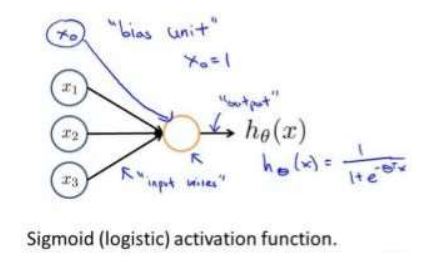Sigmoid函数在之前的逻辑回归中有写到，这里就不多写了，在上述图中，它将左边输入的和参数θ相乘后作为输入，经过自身的计算得到结果 h 0 ( x ) h_0(x) ，在神经网络中，参数又可被成为权重（weight）。

二、前向传播算法

这里直接上三层神经网络（每一层添加了偏置单元）。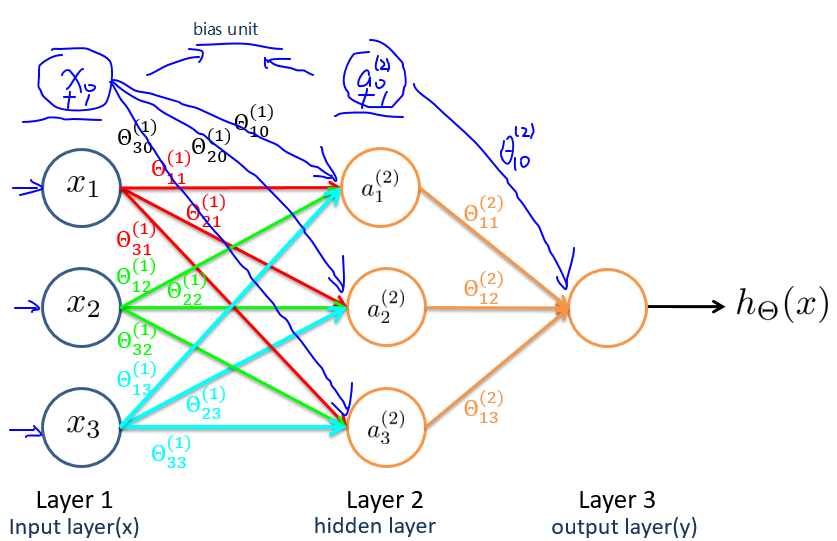x 1 , x 2 , x 3 x_1,x_2,x_3 成为输入层， a 1 , a 2 , a 3 a_1,a_2,a_3 成为隐藏层，最后一层成为输出层。

每次通过权重计算之后，需要用激活函数计算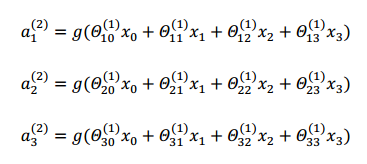θ m n i \theta_{mn}^i 表示第i层参数矩阵，m对应第i+1层激活，n对应第i层激活单元
a i ( j ) a_i^{(j)} 表示的是第i层第j个激活单元
g ( z ) g(z) 表示激活函数

输出层表达式：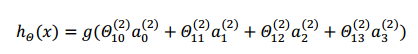从上面的图和公式可以看出，从输入层开始，不断的通过参数矩阵的运算，最后到输出层，这样的一个过程就叫前向传播算法。

神经网络和逻辑回归/线性回归的比较：
我们可以把神经网络中的隐藏层的激活单元看成是输入特征的更加高级的特征值，这些特征值可以通过之后的反向传播不断优化，调整，而逻辑回归中我们只能限制的使用原始特征（相当于神经网络的输入层）

2.反向传播算法

反向传播算法思想：

1. 将训练集数据输入到神经网络的输入层，经过隐藏层，最后达到输出层并输出结果，这是ANN的前向传播过程；
2. 由于ANN的输出结果与实际结果有误差，则计算估计值与实际值之间的误差，并将该误差从输出层向隐藏层反向传播，直至传播到输入层；
3. 在反向传播的过程中，根据误差调整各种参数的值；不断迭代上述过程，直至收敛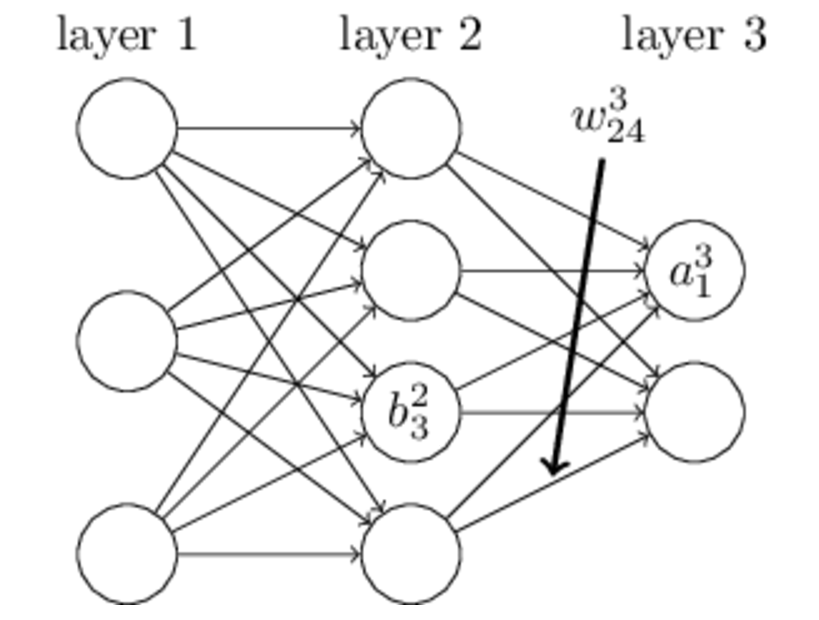ω j k l \omega_{jk}^l 表示 l − 1 l-1 层的第k个神经元连接到第 l l 层的第 j j 个神经元的额权重。
b j l b_j^l 表示第 l l 层的第 j j 个神经元的偏置
z j l z_j^l 表示第 l l 层的第 j j 个神经元的输入
z = α ω z = \alpha\omega （向量运算）
α j l \alpha_j^l 表示第 l l 层的第 j j 个神经元的输出
α = σ ( z ) \alpha=\sigma(z) σ ′ ( z ) \sigma^{'}(z) 表示激活函数）

代价函数

代价函数被用来计算神经网络输出值与实际值之间的误差。根据这个误差来调整参数。
C = 1 2 n ∑ ∥   y ( x ) − α L ( x ) ∥ 2 C = \frac{1}{2n}\sum\lVert \ y(x)-\alpha^L(x)\rVert ^2

推导过程

1. 最后一层神经网络产生的误差

δ L = ∂ C ∂ z L = ∂ C ∂ α L ∂ α L ∂ z L = ∂ C ∂ α L σ ′ ( z L ) \delta^L=\frac{\partial C}{\partial z^L}=\frac{\partial C}{\partial \alpha ^L}\frac{\partial \alpha ^L}{\partial z ^L}=\frac{\partial C}{\partial \alpha ^L}\sigma^{'}(z^L)

1. 往前推，每一层神经网络产生的误差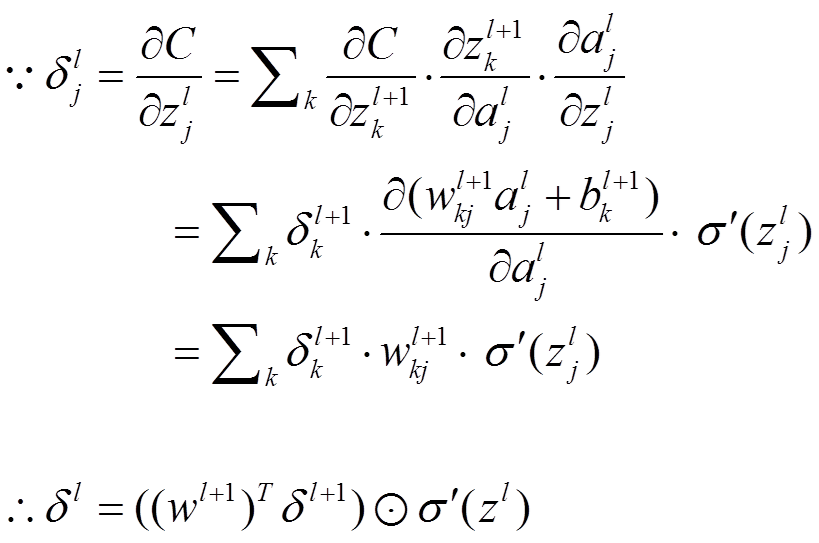2. 计算权重的梯度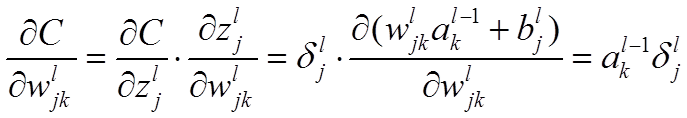代码实现

前向传播

def forward_propagate(X, theta1, theta2):
m = X.shape

a1 = np.insert(X, 0, values=np.ones(m), axis=1)
z2 = a1 * theta1.T
a2 = np.insert(sigmoid(z2), 0, values=np.ones(m), axis=1)
z3 = a2 * theta2.T
h = sigmoid(z3)

return a1, z2, a2, z3, h

反向传播

def backprop(params, input_size, hidden_size, num_labels, X, y, learning_rate):
m = X.shape
X = np.matrix(X)
y = np.matrix(y)

# reshape the parameter array into parameter matrices for each layer
theta1 = np.matrix(np.reshape(params[:hidden_size * (input_size + 1)], (hidden_size, (input_size + 1))))
theta2 = np.matrix(np.reshape(params[hidden_size * (input_size + 1):], (num_labels, (hidden_size + 1))))

# run the feed-forward pass
a1, z2, a2, z3, h = forward_propagate(X, theta1, theta2)

# initializations
J = 0
delta1 = np.zeros(theta1.shape)  # (25, 401)
delta2 = np.zeros(theta2.shape)  # (10, 26)

# compute the cost
for i in range(m):
first_term = np.multiply(-y[i,:], np.log(h[i,:]))
second_term = np.multiply((1 - y[i,:]), np.log(1 - h[i,:]))
J += np.sum(first_term - second_term)

J = J / m

# add the cost regularization term
J += (float(learning_rate) / (2 * m)) * (np.sum(np.power(theta1[:,1:], 2)) + np.sum(np.power(theta2[:,1:], 2)))

# perform backpropagation
for t in range(m):
a1t = a1[t,:]  # (1, 401)
z2t = z2[t,:]  # (1, 25)
a2t = a2[t,:]  # (1, 26)
ht = h[t,:]  # (1, 10)
yt = y[t,:]  # (1, 10)

d3t = ht - yt  # (1, 10)

z2t = np.insert(z2t, 0, values=np.ones(1))  # (1, 26)
d2t = np.multiply((theta2.T * d3t.T).T, sigmoid_gradient(z2t))  # (1, 26)

delta1 = delta1 + (d2t[:,1:]).T * a1t
delta2 = delta2 + d3t.T * a2t

delta1 = delta1 / m
delta2 = delta2 / m

delta1[:,1:] = delta1[:,1:] + (theta1[:,1:] * learning_rate) / m
delta2[:,1:] = delta2[:,1:] + (theta2[:,1:] * learning_rate) / m

# unravel the gradient matrices into a single array

展开全文weixin_43907580 2021-06-03 14:05:47
• 深度学习基础------前向传播与反向传播 深度学习

当前，深度学习已经应用到很多领域：无人驾驶汽车，黑科技以及图像分类等等，这些前沿的科技也面临许多挑战，如无人驾驶...深度学习的过程可以分为前向传播和反向传播两个过程，前向传播。 简单来说，前向传播过程就是

当前，深度学习已经应用到很多领域：无人驾驶汽车，黑科技以及图像分类等等，这些前沿的科技也面临许多挑战，如无人驾驶汽车需要进行物体的检测、行人的检测、标志的识别以及速度识别等等；图像分类已经成为一项重要技术，它是计算机视觉的核心任务，其困难之处在于图像中物体形状的改变、部分遮蔽以及背景的混入等等。让机器学习人类模拟人类大脑的思考过程，需要进行大量的实验研究才能正式投入运行，即将大量的数据分为训练集、验证集以及测试集。

深度学习的过程可以分为前向传播和反向传播两个过程，前向传播。

简单来说，前向传播过程就是数据从输入层传入，经过隐藏层，最终到达输出层的过程。如下图所示，图中包含一个输入层，一个隐藏层和一个输出层。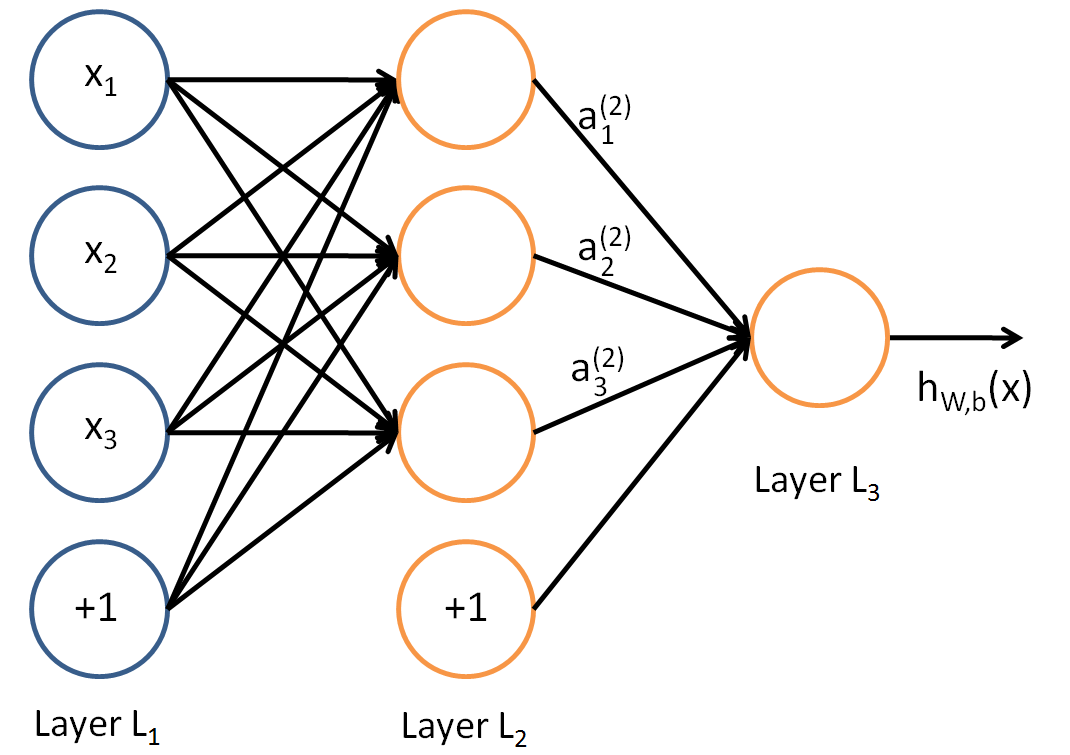对于第2层第1个节点的输出有：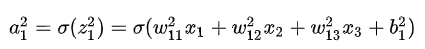对于第3层第1个节点的输出有：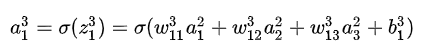而反向传播的核心在于复合函数的链式求导法则，反向传播的作用在于优化代价函数，这也是训练神经网络的目标。

加法节点

类似于2+3=5，将常数换成变量 x+y=z，如下图所示，就是一个简单的前向传播和反向传播过程（右边的图是对应反向传播过程）：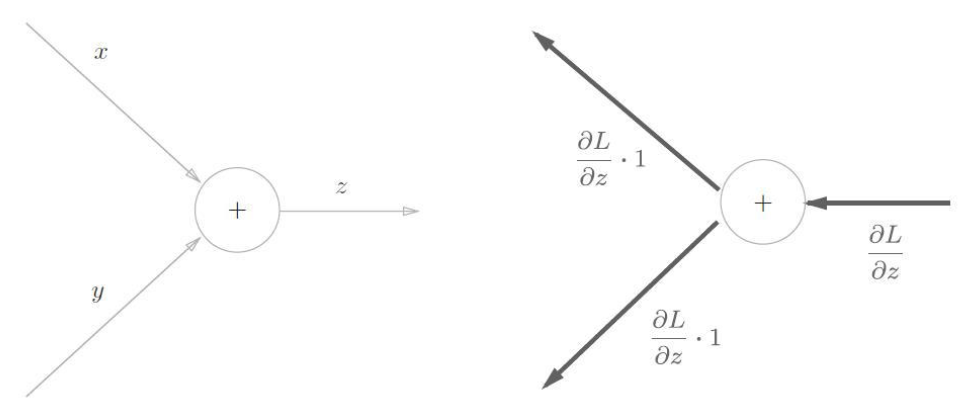乘法节点

以 z=x*y 为例，如下图所示，就是一个简单的前向传播和反向传播过程（右边的图是对应反向传播过程）：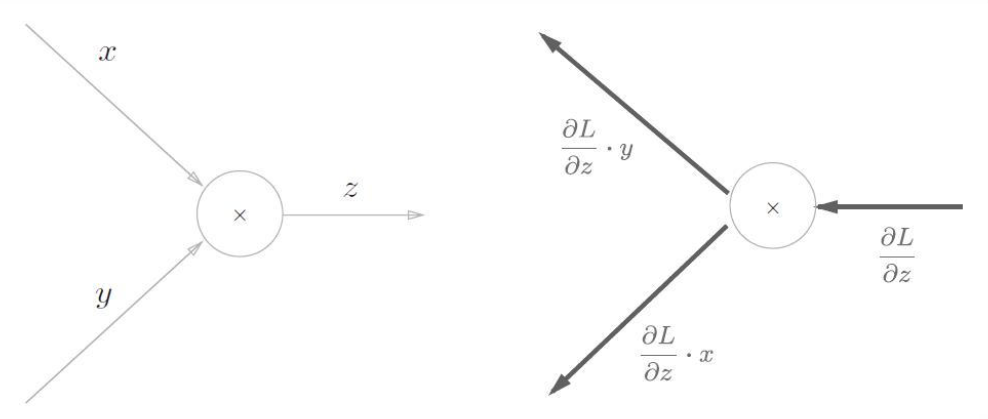加法的反向传播只是将上游的值传给下游，并不需要正向传播的输入信号。但是乘法的反向传播需要正向传播时的输入信号值。因此，实现乘法节点的反向传播时，要保存正向传播的输入信号。

从左向右进行计算是一种正方向上的传播，简称为正向传播 (forward propagation)。从右向左的传播称为反向传播 (backward propagation)。
计算图的反向传播：沿着与正方向相反的方向，乘上局部导数。
反向传播的计算顺序是：
将信号 E 乘以节点的局部导数 (∂ y/∂ x)，然后将结果传递给下一个节点。这里所说的局部导数是指正向传播中y = f (x) 的导数，也就是 y 关于 x 的导数(∂ y/∂ x)。如 y = f ( x ) = x^2 则局部导数为(∂ y/∂ x) = 2x。把这个局部导数乘以 E,然后传递给前面的节点。这就是反向传播的计算顺序。通过这样的计算，可以高效地求出导数的值，这是反向传播的要点。z=f(x,y) 求偏导数：

1）加法的偏导数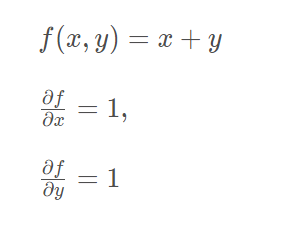2）乘法的偏导数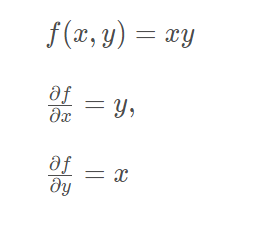举个例子：将乘法和加法组合起来。
物品a的单价是5，数量是8
物品b的单价是6，数量是5
物品的总价是多少？
5×8 + 6×5 = 70
代码如下：

class multiplication_layer:
def __init__(self):
self.x = None
self.y = None

def forward(self, x, y):
self.x = x
self.y = y
out = x * y
return out

def backward(self, d_out):
d_x = d_out * self.y
d_y = d_out * self.x
return d_x, d_y

def __init__(self):
pass

def forward(self, x, y):
out = x + y
return out

def backward(self, d_out):
d_x = d_out * 1
d_y = d_out * 1
return d_x, d_y

a_price = 5
a_num = 8
b_price = 6
b_num = 5

# layer
a_layer = multiplication_layer()
b_layer = multiplication_layer()

# forward
a_price = a_layer.forward(a_price, a_num)  # (1)
b_price = b_layer.forward(b_price, b_num)  # (2)
totoal_price = c_layer.forward(a_price, b_price)  # (3)

# backward
d_price = 1
d_a_price, d_b_price = c_layer.backward(d_price)  # (3)
d_b, d_b_num = b_layer.backward(d_b_price)  # (2)
d_a, d_a_num = a_layer.backward(d_a_price)  # (1)

print("totoal_price:", (totoal_price))
print("d_a:", d_a)
print("d_a_num:", (d_a_num))
print("d_b:", d_b)
print("d_b_num:", (d_b_num))

# totoal_price: 70
# d_a: 5
# d_a_num: 8
# d_b: 6
# d_b_num: 5

z=(x+y)^2 由两个式子构成：z = t^2 和 t = x+y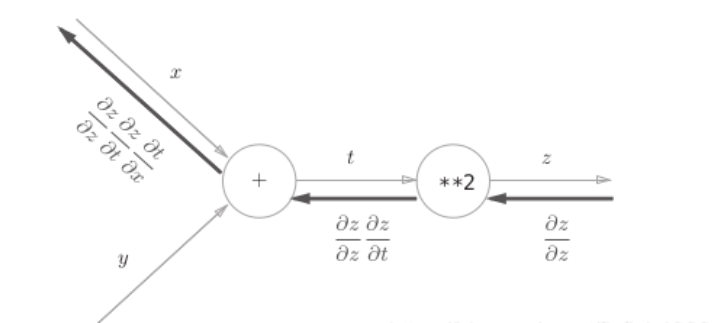简单实现一个全连接层的前向传播和反向传播
全连接层是fully connected layer，类似的名词是affine或者linear

Y = W X + B

import numpy as np
N=1
X= np.random.random((N,2))
W= np.random.random((2,3))
B= np.random.random((3,))
Y = np.dot(X, W) + B
print(Y)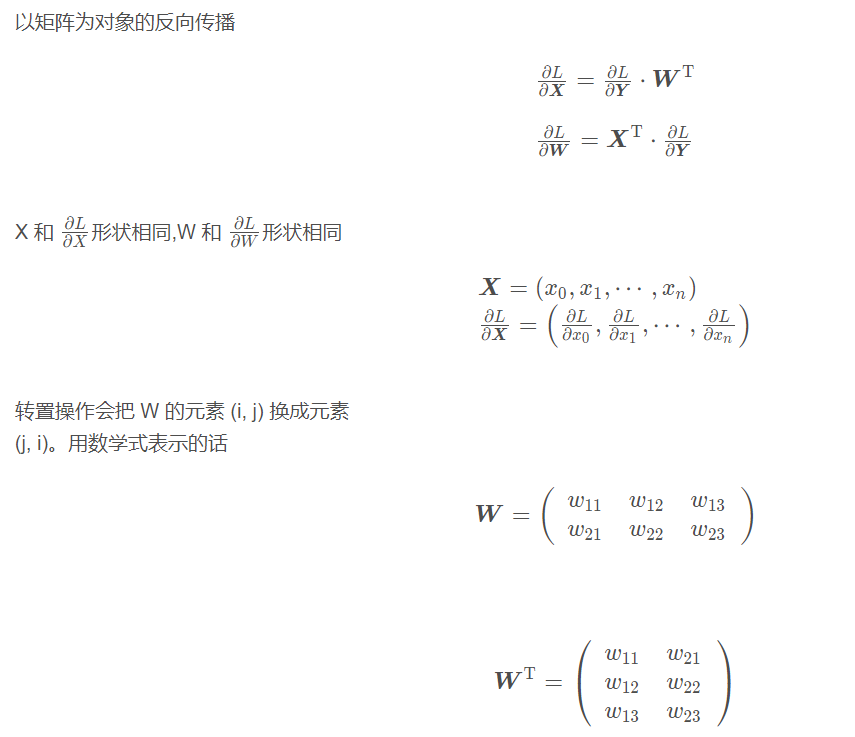import numpy as np

class fully_connected_layer:
def __init__(self,W, B):
self.X = None
self.W = W
self.B = B
self.dx = None
self.dw = None
self.db = None

def forward(self, input):
self.X = input
out = np.dot(self.X, self.W) + self.B
return out

def backward(self, d_out):
self.dx = np.dot(d_out, self.W.T)
self.dw = np.dot(self.X.T,d_out)
self.db = np.sum(d_out, axis=0)
return self.dx

np.random.seed(2020)

N=2
input= np.random.random((N,2))
weight= np.random.random((2,3))
bias= np.random.random((3,))

print(input.shape)
print("input=\n",input)

print(weight.shape)
print("weight=\n",weight)

print(bias.shape)
print("bias=\n",bias)

linear = fully_connected_layer(W=weight,B=bias)

out = linear.forward(input)
print("out=\n",out)

d_out= np.random.random(out.shape)
dx = linear.backward(d_out)
dw = linear.dw
db = linear.db
print("dx=\n",dx)
print("dw=\n",dw)
print("db=\n",db)

展开全文baidu_41774120 2021-09-29 14:29:48
• DNN的前向传播与反向传播算法 神经网络 深度学习 前向传播 后向传播

qq_23100417 2021-04-19 15:45:50
• 前向传播和后向传播 深度学习 神经网络

weixin_43464554 2021-03-27 19:16:34
• m0_56512537 2021-08-06 13:04:50
• （二十四） 手推BP神经网络的前向传播和反向传播 神经网络

m0_43609475 2021-03-11 11:22:23
• 前馈神经网络——前向传播 python tensorflow 深度学习

m0_45096530 2021-11-25 19:27:12
• u013852115 2021-06-17 16:47:05
• 机器学习二------前向传播过程 反向传播算法（BP算法）Dropout 梯度消失和梯度爆炸 html5 机器学习 html

qq_44068512 2021-09-25 18:30:34
• 【深度学习】(1) 前向传播，附python完整代码 深度学习 机器学习 python 神经网络 tensorflow

dgvv4 2021-12-04 12:06:35
• shandianfengfan 2021-04-02 00:54:14
• wdh315172 2021-04-02 11:07:30
• 源码解析] PyTorch 分布式(12) ----- DistributedDataParallel 之 前向传播 pytorch 分布式 数据并行 前向传播

weixin_47364682 2021-11-26 21:58:01
• weixin_43142450 2021-05-30 23:19:49
• 深度神经网络模型（DNN）与前向传播过程 神经网络 dnn 深度学习

qq_39691463 2021-10-15 22:03:14
• 机器学习——神经网络（前向传播） 神经网络 机器学习

weixin_43201090 2021-01-28 18:44:44
• 深度学习：神经元、神经网络、激活函数、损失函数、前向传播、后向传播 神经网络 深度学习 pytorch

haoranhaoshi 2021-09-03 21:17:25
• PyTorch入门小笔记——利用简单例子观察前向传播各个层输出的size pytorch torch 人工智能 深度学习 python

umbrellalalalala 2021-08-24 15:54:53
• 全面解析LSTM(RNN,GRU)及其前向传播过程 机器学习 深度学习

HIVAN1 2021-03-08 09:25:23
• m0_46613023 2021-01-16 22:26:32
• 前向传播与反向传播 神经网络 python 人工智能 深度学习

weixin_40604528 2021-10-17 15:30:34
• 深度学习的训练流程（前向传播，损失函数，优化器，反向传播更新w和b） 算法 深度学习 神经网络 人工智能 机器学习

qq_45234219 2021-03-16 22:13:11
• 基于tf的前向传播（张量） 机器学习

qq_46588746 2021-08-11 16:22:16
• 前向传播网络实现（类与函数）——TensorFlow2.4 网络 深度学习 keras

d8dongdong 2021-12-03 19:35:10
• pytorch中biLSTM_CRF模型源码学习（三）：前向传播中计算forward_score和gold_score 深度学习

weixin_56336619 2021-04-25 19:20:33
• 基于经典的前向传播算法和反向传播算法共同完成神经网络的训练 神经网络 深度学习 人工智能 机器学习 python

huanfeng_AI 2021-03-29 22:24:36...